Courses

# Test: Functions Of One,Two Or Three Real Variables - 5

## 20 Questions MCQ Test Topic-wise Tests & Solved Examples for IIT JAM Mathematics | Test: Functions Of One,Two Or Three Real Variables - 5

Description
This mock test of Test: Functions Of One,Two Or Three Real Variables - 5 for Mathematics helps you for every Mathematics entrance exam. This contains 20 Multiple Choice Questions for Mathematics Test: Functions Of One,Two Or Three Real Variables - 5 (mcq) to study with solutions a complete question bank. The solved questions answers in this Test: Functions Of One,Two Or Three Real Variables - 5 quiz give you a good mix of easy questions and tough questions. Mathematics students definitely take this Test: Functions Of One,Two Or Three Real Variables - 5 exercise for a better result in the exam. You can find other Test: Functions Of One,Two Or Three Real Variables - 5 extra questions, long questions & short questions for Mathematics on EduRev as well by searching above.
QUESTION: 1

Solution:
QUESTION: 2

Solution:
QUESTION: 3

### Let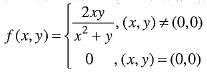then,

Solution:
QUESTION: 4

Let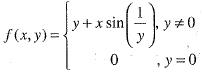then,

Solution:
QUESTION: 5

For the function
f(x, y) = x3 + y3 - 3x — 12y + 20 has,

Solution:
QUESTION: 6

The g -1({0}) for the function g(x)= ⌊x⌋ is ___________

Solution:

g({0}) for the function g(x) is {x | 0 ≤ x ≤ 1}. Put g(x) = y and find the value of x in terms of y such that [x] = y.

QUESTION: 7

The function f(x) =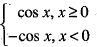has

Solution: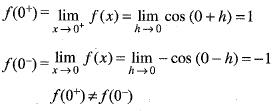irremovable discontinuity.

QUESTION: 8

If f(x) =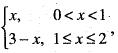then
(i)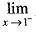f(x) = 1
(ii)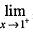f(x) = 2
(iii)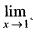f (x) =2
Q. Which of these statement(s) is/are correct?

Solution:

Given that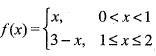Clearly , the value of function is changing at x = l, therefore, we check limit at x = l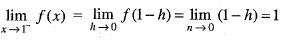and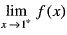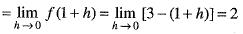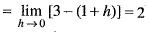QUESTION: 9

If f(x) = (x2 - 1) |x2 - 3x + 2 | + cos (|x|), then set of point of non - differentiability is

Solution:

Given that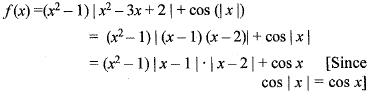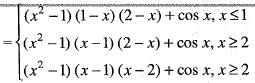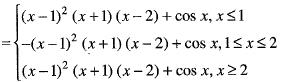Clearly, the rule of the function is changing of x = 1 and 2. So, we shall test the differentiability of f(x) only at the points x = 1, and 2
Clearly Rf'(1) = Lf'(1) and Rf'(2) ≠ Lf'(2) Hence ,f(x) is not differentiable at x = 2.

QUESTION: 10

If f(y) =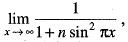then f(x) is

Solution:

Given that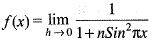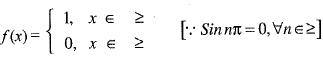Clearly f(x) is discontinuous for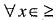QUESTION: 11

Let us consider the pair of ordered pair of sequences
(an , bn) and (cn, dn) such that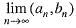= (a,b) and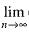(cn, dn) = (a,b), then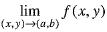does not exists, if

Solution:
QUESTION: 12

Let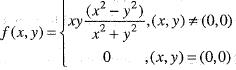then,

Solution:
QUESTION: 13

Let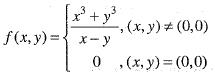then,

Solution:
QUESTION: 14

Let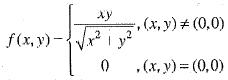then at (0, 0)

Solution:
QUESTION: 15

Let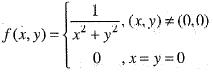then,

Solution:
QUESTION: 16

Consider the function f(x) = |x3| where x is real, then the function f (x) at y = 0 is

Solution:

Given that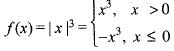It is clear that the funtion is twice differentiable at x = 0 and the other points the function is a polynomial.
Therfore, it is obviously differentiable.

QUESTION: 17

If f(x) =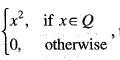then

Solution:

Given that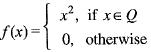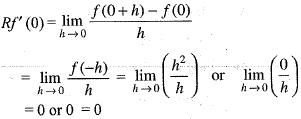Similarly, Lf'(0) = 0
Hence f(x) is differentiable at x = 0.

QUESTION: 18

Let f : [0, 10] → [0 ,10] be continuous function then

Solution:
QUESTION: 19

​The derivative of a periodic function with period T is

Solution:
QUESTION: 20

f(x) = x |x|, then choose the correct statement

Solution:

Track your progress, build streaks, highlight & save important lessons and more!

### Similar Content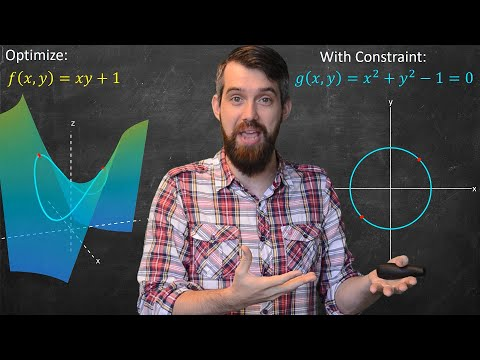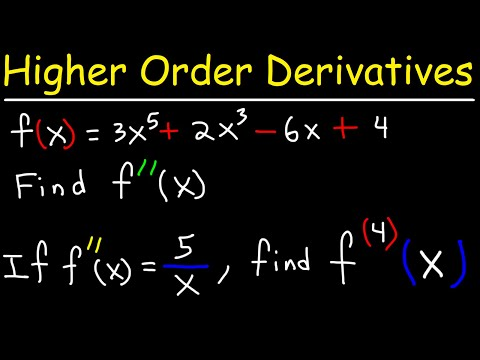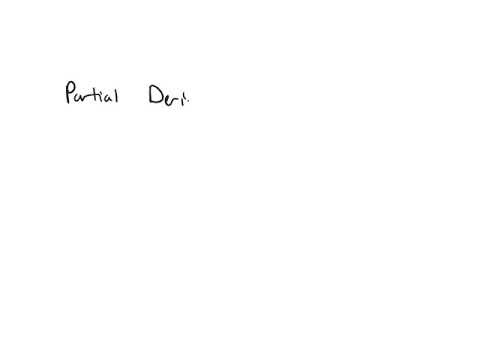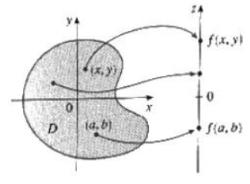### Related tests